#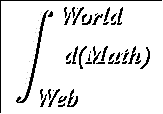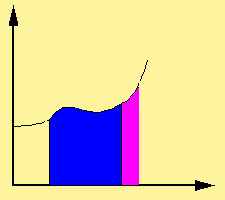Formal Definition of Limits

For a function f(x),

#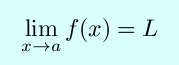if and only if for each positive number

#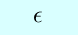(the lowercase Greek ``epsilon"), there exists a positive number

#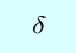(the lowercase Greek letter "delta") with the property that

#That may be quite a bit to swallow all at once. You might want to shake those epsilons and deltas from your head and take a step back. The limit is concerned with what f(x) looks like around the point x = a. The formal statement says that the limit L is the number such that if you take numbers arbitrarily close to a (or, values of x within delta of a) that the result of f applied to those numbers must be arbitrarily close to L (or, within epsilon of L).

One of the important things is that nowhere is the formal definition mention anything about the actual value of f(x) at x = a. The value of f(a) does not affect the limit, and may not even be defined.

Calculus page | World Web Math Categories Page

watko@mit.edu